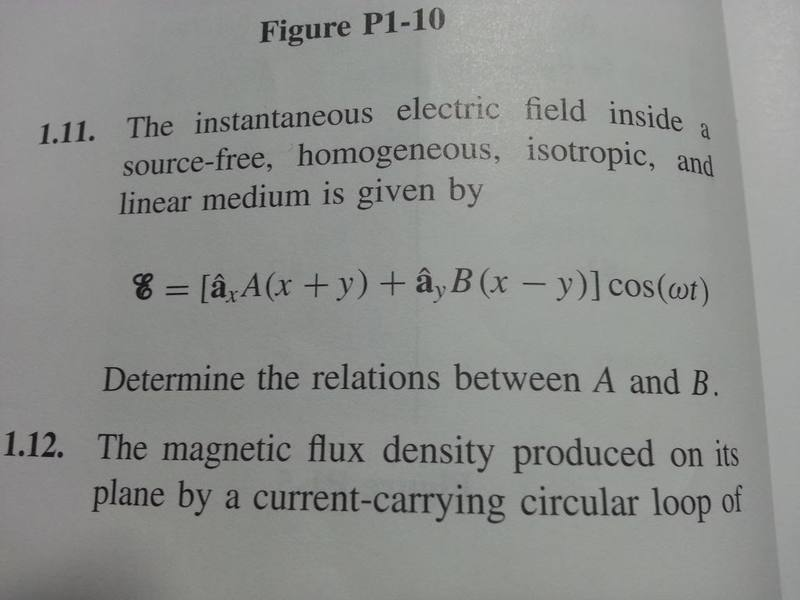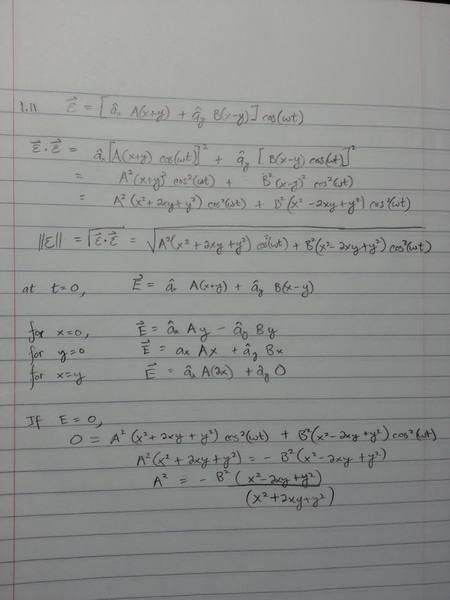# Finding the constants in an Electric Field Equation

• CanIExplore
In summary, the problem calls for finding the relationship between the constants A and B in the following equation of an instantaneous electric field. The details of the medium in which the field exists are that it is homogenous, isotropic, linear, and is source-free.f

## Homework Statement

The problem calls for finding the relationship between the constants A and B in the following equation of an instantaneous electric field:

E=[A(x+y)$\widehat{a}_{x}$+B(x-y)$\widehat{a}_{y}$]cos(wt)

The details of the medium in which the field exists are that it is homogenous, isotropic, linear, and is source-free.## The Attempt at a Solution

I really have no idea how to go about solving this problem. Initially I was thinking I was just playing around with the vector equation by seeing what it looks like when I plug in different values of x and y and when t=0 or 1. Here is what that looked like:Have you studied the properties of the "divergence" of the electric field?

The only question is, what an "instantaneous" electric field might be. I think it's just an electric field, right?

Then just use the Maxwell and constitutive equations for the given situation to constrain the functions $A$ and $B$ as much as you can.

Have you studied the properties of the "divergence" of the electric field?

And how about the curl, since the field is apparently time-invariant.

Using Maxwell's equation

$\nabla \cdot E$=$\frac{q_{ev}}{ε_{0}}$

where $q_{ev}$ is the electric charge density and noting that $q_{ev}$=0 for a source free medium, I get:

$\nabla \cdot E$={$\frac{\partial}{\partial_{x}}$$\widehat{a}_{x}$$\cdot$[$\widehat{a}_{x}$A(x+y)]+$\frac{\partial}{\partial_{y}}$$\widehat{a}_{y}$$\cdot$[$\widehat{a}_{y}$B(x-y)]}cos(ωt)=0

={$\frac{\partial}{\partial_{x}}$Ax+$\frac{\partial}{\partial_{x}}$Ay+$\frac{\partial}{\partial_{y}}$Bx-$\frac{\partial}{\partial_{y}}$By}cos(ωt)=0

(A-B)cos(ωt)=0

Which is true when ωt=$\frac{\pi}{2}$, or when A=B.

I am not really sure how to interpret this result.

Last edited:
Sorry I had some mistakes in there, I was making a few changes!

You might want to start with what you know about ##\vec{\nabla} \cdot \vec{D}## and then, as vanhees71 suggested, use the consitutive relations to relate ##\vec{D}## to ##\vec{E}##.

Using all four of the assumptions: (1) source free (2) homogeneous (3) isotropic (4) linear medium, you can deduce what ##\vec{\nabla} \cdot \vec{E}## must equal.